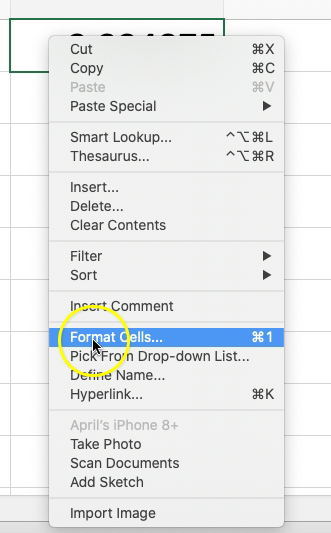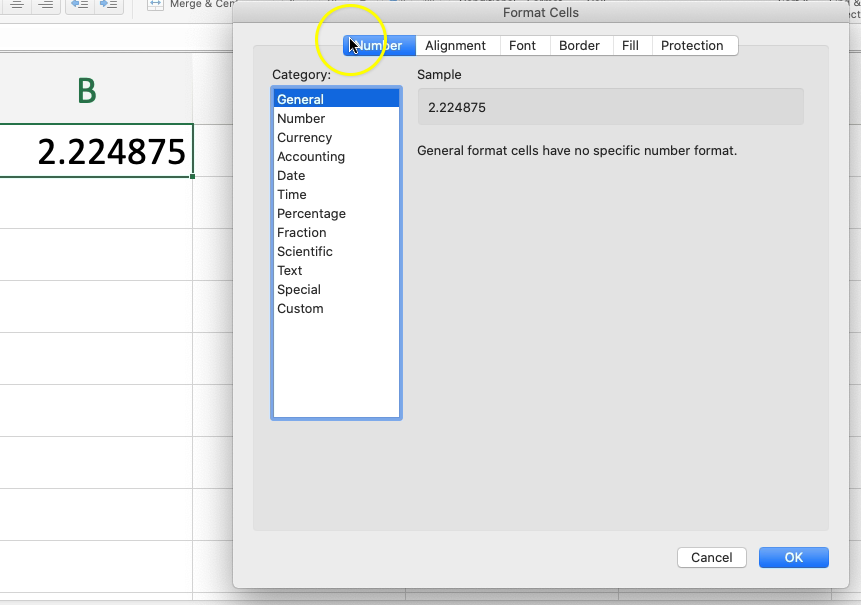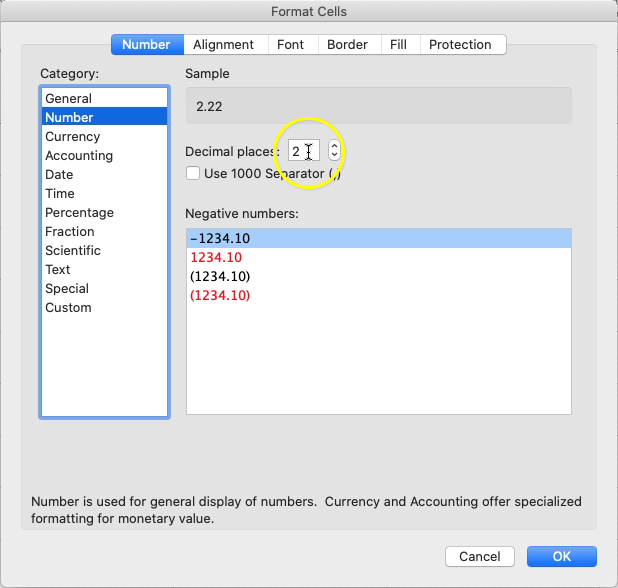Back
Rounding Using Excel
> ... Math > Excel > Rounding Using Excel

There are two ways to use this lesson. You can watch the video or just read the text below. Both will give you the same information.

Rounding Using Excel

There are several ways to round numbers using Excel. In this lesson, we cover three of those ways. You can pick the method you like the best.

1. Rounding Method #1: Format Cell
2. Rounding Method #2: Use the rounding buttons
3. Rounding Method #3: Use the ROUND function

## Rounding Method #1: Format Cell

Right click on the cell you would like to format. Select Format Cell from the drop down menu.This will open the Format Cell box. Select the number tab at the top if it isn’t already selected. You will see a list of categories on the left hand side that you can choose from.Select Number on the left hand side. This will bring up more options on the right hand side associated with the number in the cell.One of these options is to select the number of place values after the decimal point you want displayed in the cell.

Changing this will not change the full value of the number in the cell. You will still be able to see the original value of the number in the cell by clicking on the cell and looking in the formula bar.

## Rounding Method #2: Use the Rounding Buttons

In the Home toolbar there are two buttons used to quickly round numbers within cells. The button to increase decimal places has several three zeros and an arrow pointing left. The button to decrease decimal places has three zeros and an arrow pointing to the right.

To use these rounding buttons you must first select the cell(s) you want to edit and then select the rounding button of choice. Each time you select the rounding button it changes the decimal places by one place.

Example:

If your cell contained the number 3.8495 and you wanted to round to the nearest tenth, you would need to select the cell and then click the decrease decimal button three times.

• The first click would display the number 3.850. (The 9 in the thousandths place would round up to a 10 and carry the 1 into the hundredths place.)
• The second click would display the number 3.85.
• The third click would display the number 3.8. (Note: This did not round up to 3.9 because if we look at the original number, there is a 4 in the hundredths place, so rounding to the tenths place would not change the 8 to a 9. Excel looks at the original number when rounding and not the previously rounded number.)

## Rounding Method #3: Use the ROUND Function

Type into the cell “=round(”.

There are two arguments in this function.

1. The original number you want to round. This can also be a referenced cell.
2. The number of decimal place values to round to. If you want to round to the tenths place, then type in 1. To round to the hundredths place, type in 2. You can also use this to round to place values before the decimal point such as the tens or hundreds place. To do this use negative numbers for the place value.

Example: Round 4.87361 to the nearest hundredths place. Type “=round(4.87361,2)” into the cell. (Don’t include the quotation marks.) It will display 4.87.
Example: Round 4897.29 to the nearest hundreds place. Type “=round(4897.29,-2)” into the cell. (Don’t include the quotation marks.) It will display 4900.

## Need More Help?

1. Study other Math Lessons in the Resource Center.
2. Visit the Online Tutoring Resources in the Resource Center.